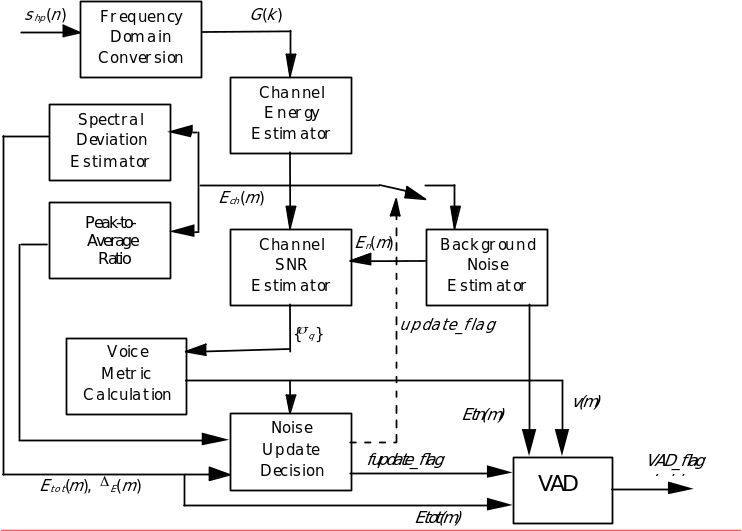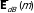## 4.3 Functional description

06.943GPPTSVoice Activity Detector (VAD) for Adaptive Multi Rate (AMR) speech traffic channels

The block diagram of the VAD algorithm is depicted in figure 1. The VAD algorithm uses parameters of the speech encoder to compute the Boolean VAD flag (VAD_flag).Figure 4.1: Block Diagram of the VAD algorithm: Option 2

Input:

– The output of the High-Pass Filter, {shp(n)}

– LTP_flag is generated by the comparison of the long-term prediction gain to a constant threshold LTP_THLD, where the long-term prediction gain is derived from the speech encoder open-loop pitch predictor.

Output:

– The output of the vad is designated as VAD_flag

Initialization:

The following variables shall be set to zero at initialization (frame m = 0):

– The pre-emphasis memory

The following shall be initialized to a startup value other than zero:

– The channel energy estimate, Ech(m), (see Section 4.3.2);

– The long-term power spectral estimate,, (see Section 4.3.5);

– The channel noise estimate, En(m), (see Section 4.3.8).

Processing: The following procedures shall be executed two times per 20 ms speech frame and the current 10 ms subframe shall be denoted m.